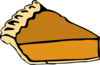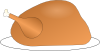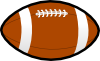# Thanksgiving Problem Set

By Renee | Added Nov 25, 2014Want to test your math skills and get into the Thanksgiving spirit? Try our Thanksgiving word problems!

## Lower Elementary:

Question: At a Thanksgiving dinner, 6 relatives are visiting from Nevada, 7 relatives are visiting from California, and 4 relatives are visiting from Arizona. How many total relatives are visiting?

Solution: To find the number of relatives visiting, we need to add the members visiting from the different states. So, we need to add 6 + 7 + 4. Notice that if we add 6 + 4 first, we would get 10. So, 6 + 7 + 4 = 10 + 7 = 17. There are 17 relatives visiting.## Upper Elementary:

Question: The Morgans had pumpkin pie for their Thanksgiving dessert. The mom ate 1/4 of the pie. The dad ate 2/3 of what was remaining after the mom ate her portion. The daughter ate 1/2 of what was remaining after the dad ate his portion. How much of the pie is left after the daughter ate her portion?

Solution: From the whole pie, the mom ate 1/4 of it. 1 - 1/4 = 3/4. There is 3/4 of the pie left after the mom ate her portion. The dad ate 2/3 of what was remaining. Breaking 3/4 into 3 equal parts, each part is equal to 1/4. If 1/3 of 3/4 is 1/4, then 2/3 of 3/4 is 2/4 = 1/2. So the dad ate 1/2 of the pie. This means that there is 3/4 - 1/2 = 1/4 of the pie left for the daughter. The daughter ate half of what was remaining. Half of 1/4 is 1/8. There is 1/8 of the pie left after the daughter ate her portion.## Middle School:

Question: Right before Thanksgiving, a turkey went on sale from \$20 to \$17. What was the amount of the discount?

Solution: To find the percent discount, find the difference between the original and sale price and divide by the original price. \$20 - \$17 = \$3. Now, we have 3/20. Since percent means for each hundred, one way to find the percent is to make the denominator equal to 100. 3/20 = 15/100. So, the turkey is on sale at a 15% discount.

## Algebra and Up:Question: The Goldmans are playing a game of football after their Thanksgiving meal. Bob threw the football and its path can be traced by the function h(t) = -t2 + 3t + 10 where t is the time from when Bob threw the football in seconds. At what time will the football hit the ground?